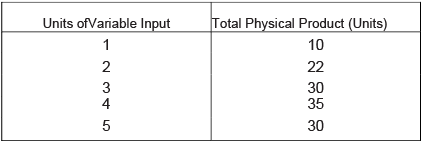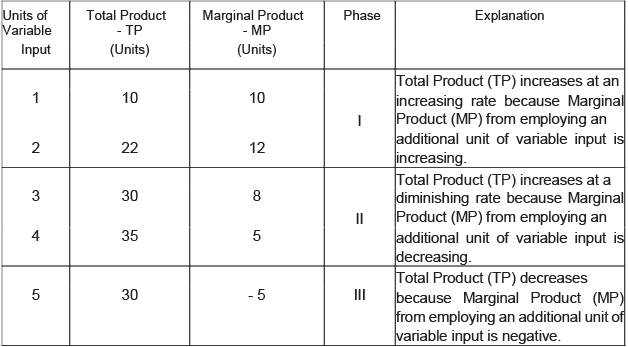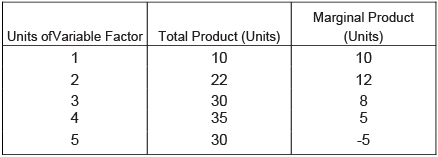Courses

# Long Questions With Answers - Production And Costs Commerce Notes | EduRev

## Commerce : Long Questions With Answers - Production And Costs Commerce Notes | EduRev

The document Long Questions With Answers - Production And Costs Commerce Notes | EduRev is a part of the Commerce Course Economics Class 11.
All you need of Commerce at this link: Commerce

Q.1. Giving reasons, state whether the following statements are true or false:
(i) Average Costs fall only when Marginal Cost falls
(ii) The difference between Average Total Cost and Average Variable Cost is constant
(iii) When Total Revenue is maximum, Marginal Revenue is also maximum.
Ans.
(i) False. Marginal Cost curve always cuts the Average Cost curve from below at its minimum point. This implies that MC increases even when Average Cost is falling.
(ii) False. The difference between Average Total Cost and Average Variable Cost is Average Fixed Cost. Average Fixed Cost (AFC) goes on declining as the level of output increases because Fixed Cost is constant at every level of output. Thus, the difference between Average Total Cost and Average Variable Cost is not constant, but declining.
(iii) False. When Total Revenue is maximum then Marginal Revenue is zero.

Q.2. Giving reasons, state whether the following statements are true or false:
(i) When there are diminishing returns to a factor, Marginal Product and Total Product both always diminish
(ii) When Marginal Revenue is positive and constant,Average and Total Revenue will both increase at constant rate
(iii) As output is increased, the difference between Average Total Cost and Average Variable Cost falls and ultimately become zero.
Ans.
(i) False. When there are diminishing returns to factor then Marginal Product decreases, but Total Product increases at a decreasing rate.
(ii) False. When Marginal Revenue is positive and constant then Average Revenue is also positive and constant. In this situation, only Total Revenue increases at constant rate.
(iii) False. The difference between Average Total Cost and Average Variable Cost falls is Average Fixed Cost. Average Fixed Cost (AFC) goes on declining as the level of output increases because Fixed Cost is constant at every level of output. However, it never becomes zero. Thus, the difference between Average Total Cost and Average Variable Cost falls as output is increased, but it never becomes zero.

Q.3. State whether the following statements are true or false. Give reasons for your answer.
(i) When there are diminishing returns to a factor Total Product first increases and then starts falling
(ii) When Marginal Revenue falls to zero, Average Revenue becomes maximum
(iii) The difference between Total Cost and Total Variable Cost falls with increase in output
Ans.
(i) True. When there are diminishing returns to a factor, TP first increases and then starts falling. Diminishing returns to a factor operates when MP starts falling. Thus, TP, in this case, increases at a diminishing rate. When MP becomes negative, TP starts falling.
(ii) False. When Marginal Revenue falls to zero, Total Revenue becomes maximum. In this situation, Average Revenue continues to fall.
(iii) False. The difference between Total Cost and Total Variable Cost is Total Fixed Cost. The difference between and Total Fixed Cost remains constant, no matter what the level of output is. Thus, the difference between Total Cost and Total Variable Cost also remains constant.

Q.4. Identify the three phases of the Law of Variable Proportions from the following and also give reason behind each phase:Ans.
The factors of production can be classified as fixed and variable factors. The fixed factors cannot be changed over a short period. Law of Variable Proportions states that when more and more units of a variable factor are employed, keeping the other factors fixed, the Total Product (TP) first increases at an increasing rate, then increases at a diminishing rate and finally starts falling. The law explains the change in output due to the change in the proportions between the fixed and variable factors. The law can be explained with the help of the following schedule.Q.5. Explain the law of returns to a factor with the help of Total Product and Marginal Product schedule.
Ans.
Returns to a factor means the increase in the Total Product (TP) per unit increase in the employment of a variable factor, when the employment of other factors is kept constant. The law of returns to a factor can be explained with the help of following schedule:It is clear from the schedule that when MP increases from 10 to 12 units, TP also increases at an increasing rate. This stage is known as increasing returns to a factor. When MP decreases from 12 to 8 to 5 units, TP increases at a diminishing rate. This stage is known as diminishing returns to a factor. When MP decreases from 5 units to -5 units then TP also decreases from 35 to 30 units. This stage is known as negative returns to a factor.

Offer running on EduRev: Apply code STAYHOME200 to get INR 200 off on our premium plan EduRev Infinity!

,

,

,

,

,

,

,

,

,

,

,

,

,

,

,

,

,

,

,

,

,

;